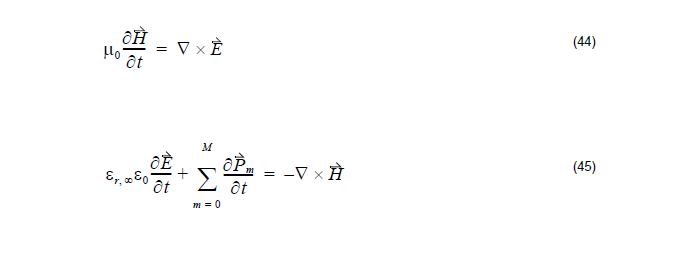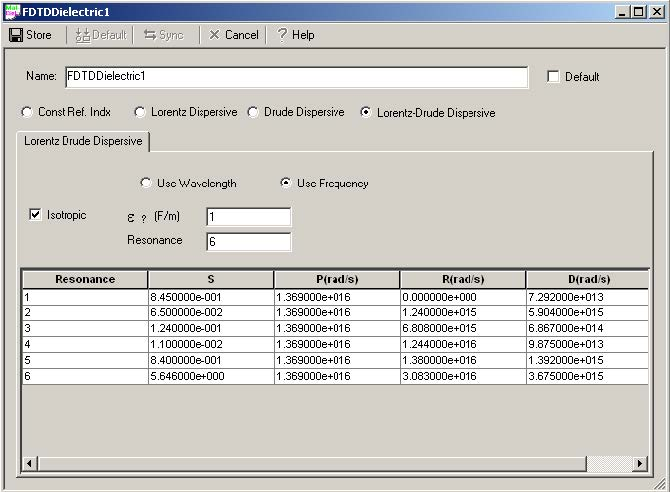The Lorentz-Drude model in (43) is in the frequency domain. However, FDTD is a time
domain method and therefore would be suitable for broadband simulations. We need
to transform (43) to time domain so that FDTD can handle the fullwave-analysis for
the Lorentz-Drude material. This transformation to time domain is accomplished by
using the Polarization philosophy within Maxwell’s equation. The Lorentz-Drude
model in time domain can be expressed:The FDTD algorithm can be derived based on the above equation.

## Lorentz-Drude model user interface in OptiFDTDFigure 7: Lorentz-Drude material definition data entry interface

where:

• S = Strength of the corresponding resonance terms
• P (rad/s) = Plasma frequency
• R (rad/s) = Resonant frequency
• D (rad/s) = Collision frequency (or damping factor )